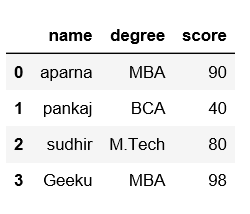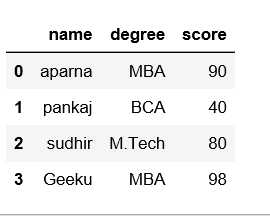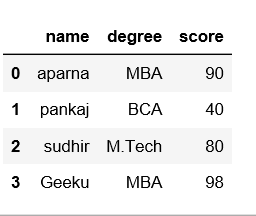# Saving a Pandas Dataframe as a CSV

Pandas is an open source library which is built on top of NumPy library. It allows user for fast analysis, data cleaning & preparation of data efficiently. Pandas is fast and it has high-performance & productivity for users.

Most of the datasets you work with are called DataFrames. DataFrames is a 2-Dimensional labeled Data Structure with index for rows and columns, where each cell is used to store a value of any type. Basically, DataFrames are Dictionary based out of NumPy Arrays.

Let’s see how to save a Pandas DataFrame as a CSV file using `to_csv()` method.

Example #1: Save csv to working directory.

 `# importing pandas as pd  ` `import` `pandas as pd  ` `  `  `# list of name, degree, score ` `nme ``=` `[``"aparna"``, ``"pankaj"``, ``"sudhir"``, ``"Geeku"``] ` `deg ``=` `[``"MBA"``, ``"BCA"``, ``"M.Tech"``, ``"MBA"``] ` `scr ``=` `[``90``, ``40``, ``80``, ``98``] ` `  `  `# dictionary of lists  ` `dict` `=` `{``'name'``: nme, ``'degree'``: deg, ``'score'``: scr}  ` `    `  `df ``=` `pd.DataFrame(``dict``) ` ` `  `# saving the dataframe ` `df.to_csv(``'file1.csv'``) `

Output:Example #2: Saving CSV without headers and index.

 `# importing pandas as pd  ` `import` `pandas as pd  ` `  `  `# list of name, degree, score ` `nme ``=` `[``"aparna"``, ``"pankaj"``, ``"sudhir"``, ``"Geeku"``] ` `deg ``=` `[``"MBA"``, ``"BCA"``, ``"M.Tech"``, ``"MBA"``] ` `scr ``=` `[``90``, ``40``, ``80``, ``98``] ` `  `  `# dictionary of lists  ` `dict` `=` `{``'name'``: nme, ``'degree'``: deg, ``'score'``: scr}  ` `    `  `df ``=` `pd.DataFrame(``dict``) ` ` `  `# saving the dataframe ` `df.to_csv(``'file2.csv'``, header``=``False``, index``=``False``) `

Output:Example #3: Save csv file to a specified location.

 `# importing pandas as pd  ` `import` `pandas as pd  ` `  `  `# list of name, degree, score ` `nme ``=` `[``"aparna"``, ``"pankaj"``, ``"sudhir"``, ``"Geeku"``] ` `deg ``=` `[``"MBA"``, ``"BCA"``, ``"M.Tech"``, ``"MBA"``] ` `scr ``=` `[``90``, ``40``, ``80``, ``98``] ` `  `  `# dictionary of lists  ` `dict` `=` `{``'name'``: nme, ``'degree'``: deg, ``'score'``: scr}  ` `    `  `df ``=` `pd.DataFrame(``dict``) ` ` `  `# saving the dataframe ` `df.to_csv(r``'C:\Users\Admin\Desktop\file3.csv'``, index``=``False``) `

Output:Example #4: Write a DataFrame to CSV file using tab separator.

 `import` `pandas as pd ` `import` `numpy as np ` `users ``=` `{``'Name'``: [``'Amit'``, ``'Cody'``, ``'Drew'``], ` `     ``'Age'``: [``20``,``21``,``25``]} ` `df ``=` `pd.DataFrame(users, columns``=``[``'Name'``,``'Age'``])``#create DataFrame ` `print``(``"Original DataFrame:"``) ` `print``(df) ` `print``(``'Data from Users.csv:'``) ` `df.to_csv(``'Users.csv'``, sep``=``'\t'``, index``=``False``,header``=``True``) ` `new_df ``=` `pd.read_csv(``'Users.csv'``) ` `print``(new_df) `

Output:

```Original DataFrame:
Name  Age
0  Amit   20
1  Cody   21
2  Drew   25
Data from Users.csv:
Name\tAge
0  Amit\t20
1  Cody\t21
2  Drew\t25
```

My Personal Notes arrow_drop_upIf you like GeeksforGeeks and would like to contribute, you can also write an article using contribute.geeksforgeeks.org or mail your article to contribute@geeksforgeeks.org. See your article appearing on the GeeksforGeeks main page and help other Geeks.

Please Improve this article if you find anything incorrect by clicking on the "Improve Article" button below.

Improved By : gauravbabbar25

Article Tags :

4

Please write to us at contribute@geeksforgeeks.org to report any issue with the above content.